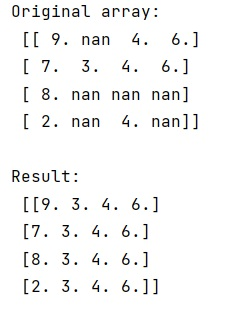# Replace NaN values with average of columns in NumPy array

Given a NumPy array, we have to replace NaN values with average of columns it.
Submitted by Pranit Sharma, on February 15, 2023

NumPy is an abbreviated form of Numerical Python. It is used for different types of scientific operations in python. Numpy is a vast library in python which is used for almost every kind of scientific or mathematical operation. It is itself an array which is a collection of various methods and functions for processing the arrays.

## NumPy Array - Replace NaN values with average of columns

Suppose that we are given a numpy ndarray that contains some nan values, we need to replace each nan value with the average of its column.

While creating a DataFrame or importing a CSV file, there could be some NaN values in the cells. NaN values mean "Not a Number" which generally means that there are some missing values in the cell.

For this purpose, we will first find the mean of those columns where nan is present and then we will find the indices where nan is present. Finally, we will place the column mean values at the specified indices using arr.take() method.

Let us understand with the help of an example,

## Python code to replace NaN values with average of columns in NumPy array

```# Import numpy
import numpy as np

# Creating a numpy array
arr = np.array([
[ 9,np.nan ,4,6],
[7, 3, 4, 6],
[ 8,np.nan ,np.nan,np.nan],
[ 2,np.nan ,4,np.nan]])

# Display original array
print("Original array:\n",arr,"\n")

# getting mean of columns where nan is present
mean = np.nanmean(arr, axis=0)

# Getting indices of nan
ind = np.where(np.isnan(arr))

# Assigning mean values in place of nans
arr[ind] = np.take(mean, ind)

# Display result
print("Result:\n",arr)
```

Output: